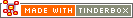^mean( group , attribute[, precision] )^Code Type: Calculation Code Scope of Action: group

^mean( group , attribute[, precision] )^

Returns the arithmetical mean attribute value for all the values of the notes within the group.

An optional third argument lets you set the number of decimal points that are exported by the numeric group mark-up elements(^sum^, ^min^, ^max^, ^mean^, ^count^). Note that the precision is always the third argument.

 Up: Calculation Previous: ^max( group , attribute[, precision] )^ Next: ^min( group , attribute[, precision] )^

[Last updated: 14 Dec 2009, using v5.0]